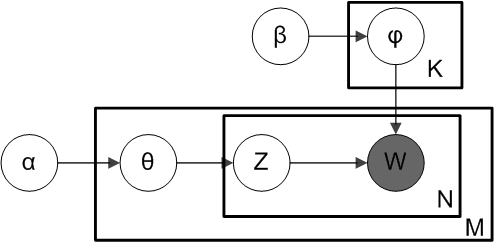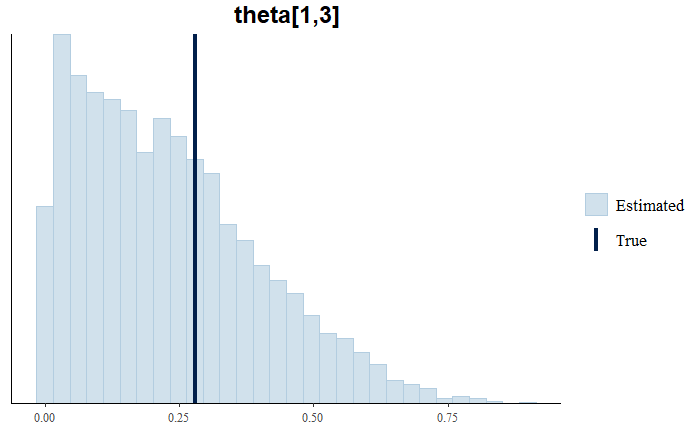Search
•Mithila Guha

# LDA Model: Simulated Data Generation in R & Parameter Recovery Study in RStan

In this post, I explain how to replicate the LDA model of Jacobs, Donkers, and Fok (2016) using Stan. They adapted the standard LDA model of Blei et al. (2003) and evaluated the model for purchase prediction in large assortments using purchase history data.

In standard LDA setup, documents are probability distributions over latent topics and topics are probability distributions over words. Jacobs, Donkers, and Fok applied the standard LDA model in the purchase prediction context using the following adjustments: topics = motivations, words= products, document= purchase. Let's take a look at how we can replicate the LDA model of their paper in R.

Before we fit any model to a real dataset, it is helpful to simulate data according to the model using known (fixed) parameter values and to see if these "true" parameter values are (approximately) recovered by fitting the model to the simulated data. At first, we need to set a working directory and install the necessary packages.

```setwd("C:\\Users\\mithi\\Desktop\\LDAstan")
library(rstan)
library(gdata)
library(bayesplot)
library(gtools)```

First Step:

a) Generating Simulated LDA Data: We will set up how many topics, words, documents we want to work with.

```options(scipen = 999) # disabling scientific notation
K <-5 #number of topics/motivation
V <-15 #number of words/products
D <-20 #number of doc/purchase history of individuals
avg_doc_length <- 300```

In the LDA model, the document length is modeled as a Poisson distribution, so I am setting the document length accordingly and find the total number of purchased products by summing up.

```doc_length <- rpois(D,avg_doc_length)
N <- sum(doc_length)#total word instances/total number of purchased products
w <- rep(NA,N) #represents a particular word n
doc <- rep(NA,N)#represents a particular document id for word n  ```

The next step is to set up the α and 𝛽 shape parameters of a Dirichlet distribution. According to the LDA, we need to draw a random sample representing the topic distribution, or topic mixture, of a particular document from a Dirichlet distribution Dir(α). α is the parameter of the Dirichlet prior for per-document topic distribution. This topic distribution is θ, where, θ~Dirichlet(α). From θ, we are going to select a particular topic Z based on the distribution.Next, we are going to set α and draw θ from the Dirichlet distribution Dir(α).

```alpha<-runif(K, min=0.5, max=1) #making it asymmetric
theta<-rdirichlet(D, alpha)```

Next, from another Dirichlet distribution Dir(𝛽), where 𝛽 is the parameter of the Dirichlet Prior for per topic word distribution, which has different distributions for words for a topic. We select a random sample representing the word distribution of the topic Z. This word distribution is φ. From φ, we choose the word W.

```beta <- rep(200/V,V) #making it symmetric too
phi <- array(NA,c(5,15)) # 5 topics, 15 words
phi<-rdirichlet(K, beta) ```

In standard LDA setup: topic z comes from a mixture of topics for a single document, each z follows a multinomial distribution of θ. Word n follows a multinomial distribution of φ, there are many mixtures of words for topic z, which mixture I am picking comes from a multinomial distribution. Next, I will be generating data for each word and document.

```n <- 1
for (d in 1:D) {
for (i in 1:doc_length[d]) {
z <- which(rmultinom(1,1,theta[d,]) == 1)
w[n] <- which(rmultinom(1,1,phi[z,]) == 1)
doc[n] <- d
n <- n + 1
}
}     ```

Next, I will save the generated data as a ".Rdata file" and save the dataset to use later.

```data <- list(K=K, V=V, D=D, N=N, z=z, w=w, doc=doc, alpha=alpha, beta=beta)
save(data, file = "data_LDA2.RData") dump(c("K","V","D","N","z","w","doc", "alpha", "beta"),"lda.RData");```

b) Specify The LDA Model in Stan: Now we can fit the model in Stan using the simulated data set we just created. The first step is specifying the Stan code for the LDA model:

```model.code = "
data {
int<lower=2> K;       // num topics(for us, motivation)
int<lower=2> V;       // num words, products
int<lower=1> D;       // num docs, number of purchase histories
int<lower=1> N;       // total word instances, total product instance
int<lower=1,upper=V> w[N];    // word n or product n
int<lower=1,upper=D> doc[N];  // doc ID for word n or CustomerID
vector<lower=0>[K] alpha;     // topic prior
vector<lower=0>[V] beta;      // word prior
}
parameters {
simplex[K] theta[D];   // topic dist for doc d
simplex[V] phi[K];     // word dist for topic k
}
model {
for (d in 1:D)
theta[d] ~ dirichlet(alpha);  //topic dist for doc d
for (k in 1:K)
phi[k] ~ dirichlet(beta);     // word dist for topic k
for (n in 1:N) {
real gamma[K];
for (k in 1:K)
gamma[k] = log(theta[doc[n], k]) + log(phi[k, w[n]]);
target += log_sum_exp(gamma);  //in mixture models, the log-sum-of-exponents function is used to stabilize the numerical arithmetic;
}
}
"#close quote for model string```

We have now saved the Stan code in a string object called model.code. We use Stan to estimate the model with the following R code:

```options(mc.cores=4) //to run parallel chains
stan.model <- stan_model(model_code = model.code)
fit1 <- sampling(stan.model, data = data, iter = 2000, chains = 3, verbose = TRUE, warmup = 1000, control = list(adapt_delta = 0.99))```

Next, I will print the fit1 values to see the details of the effective sample size (n_eff ), and the R-hat statistic (Rhat). For each parameter, n_eff is a crude measure of effective sample size, Rhat is the potential scale reduction factor on split chains (at convergence, Rhat=1).

`print(fit1, pars = "theta")`

Let's take a look at the posteriors for the first 10 parameters for theta. The Rhat values for all the parameters are close to 1, and n_eff values are also high.

```
mean se_mean  sd  2.5%  25%   50%   75%   97.5% n_eff Rhat
theta[1,1]   0.17  0.00   0.15 0.00  0.04  0.13  0.26   0.54  1777 1.00
theta[1,2]   0.16  0.00   0.15 0.00  0.04  0.12  0.24   0.54  2182 1.00
theta[1,3]   0.23  0.00   0.17 0.01  0.09  0.21  0.33   0.61  1392 1.01
theta[1,4]   0.23  0.00   0.17 0.01  0.10  0.20  0.34   0.61  1396 1.00
theta[1,5]   0.21  0.00   0.16 0.00  0.08  0.18  0.32   0.59  1955 1.00
theta[2,1]   0.17  0.00   0.17 0.00  0.03  0.11  0.24   0.61  1409 1.00
theta[2,2]   0.15  0.00   0.16 0.00  0.03  0.09  0.23   0.58  1117 1.00
theta[2,3]   0.24  0.01   0.20 0.01  0.08  0.19   0.36   0.71  929 1.01
theta[2,4]   0.23  0.01   0.18 0.01  0.08  0.19   0.34   0.66  997 1.00
theta[2,5]   0.21  0.01   0.18 0.00  0.06  0.17  0.31   0.67  1032 1.00

```

c) Model Checking: Now, let's conduct a visual posterior analysis from our parameter recovery study to see the fit of the model. We will be generating plots comparing MCMC estimates to the "true" parameter values.

```install.packages("bayesplot")
install.packages("coda")
library(bayesplot)
library(coda)
library(rstan)

# histograms of parameter draws with true value added as vertical line
mcmc_recover_hist(As.mcmc.list(fit1,pars=c("theta[1,1]")), true=0.00505664)
mcmc_recover_hist(As.mcmc.list(fit1,pars=c("theta[1,2]")), true=0.1586917)
mcmc_recover_hist(As.mcmc.list(fit1,pars=c("theta[1,3]")), true=0.2788084)
#mcmc_trace(fit1,pars=c("phi[2,2]"))
mcmc_recover_hist(As.mcmc.list(fit1,pars=c("phi[1,1]")), true=0.05397605)
mcmc_recover_hist(As.mcmc.list(fit1,pars=c("phi[1,2]")), true=0.07472266)
mcmc_recover_hist(As.mcmc.list(fit1,pars=c("phi[1,3]")), true=0.06185119)```

From the plots, we see that the true value is within the posterior distribution:This summarizes how to generate simulated data from the LDA model and run a parameter recovery study in Stan to see the fit of the model.